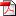# Did you realise that it is dangerous to assume data are interval scale?

Many machine learning algorithms make an implicit assumption that numeric data are interval scale, specifically, that a unit difference between values has the same meaning irrespective of the magnitude of those values. For example, most distance measures would rate values of 19 and 21 to both be the same distance from a value of 20.  However, suppose the three values are measures of miles per gallon.  It may be arbitrary whether the variable in question was recorded as mile per gallon or gallons per mile.  If they had been expressed as the latter, they would be 0.0526, 0.5000 and 0.0476.  The value corresponding to 20 (0.5000) would be closer to the value corresponding to 21 (0.0476) than to the value corresponding to 19 (0.0526).

Examples of learning algorithms that make this assumption include any that use conventional distance measures and most linear classifiers.

Our studies  have shown that for tasks as diverse as information retrieval and clustering, applying transformations to the data, such as replacing values by their square root or natural logarithm, often improves performance, indicating that the interval scale assumption is not justified.

A weaker assumption than the interval scale assumption is that numeric data are ordinal.  Under this assumption, order matters, but the magnitudes of differences in values are not specified.  Hence, for ordinal data, we can assert that 21 is more similar to 20 than 19, but not that 21 is more similar to 20 than is 18.  Our studies have shown that converting data to ranks often improves performance across a range of machine learning algorithms .

However, conversion to ranks entails a significant computational overhead if a learned model is to be applied to unseen data.  Mapping a new value onto a rank in a training set is an operation of order log training set size.  In consequence, it can be advantageous to use algorithms that assume only that data are ordinal scale, as do decision trees and algorithms built thereon, such as random forests.

### ReferenceFernando, T. L., & Webb, G. I. (2017). SimUSF: an efficient and effective similarity measure that is invariant to violations of the interval scale assumption. Data Mining and Knowledge Discovery, 31(1), 264-286.
[Bibtex]
``````@Article{FernandoWebb16,
Title = {SimUSF: an efficient and effective similarity measure that is invariant to violations of the interval scale assumption},
Author = {Fernando, Thilak L. and Webb, Geoffrey I.},
Journal = {Data Mining and Knowledge Discovery},
Year = {2017},
Number = {1},
Pages = {264-286},
Volume = {31},
Abstract = {Similarity measures are central to many machine learning algorithms. There are many different similarity measures, each catering for different applications and data requirements. Most similarity measures used with numerical data assume that the attributes are interval scale. In the interval scale, it is assumed that a unit difference has the same meaning irrespective of the magnitudes of the values separated. When this assumption is violated, accuracy may be reduced. Our experiments show that removing the interval scale assumption by transforming data to ranks can improve the accuracy of distance-based similarity measures on some tasks. However the rank transform has high time and storage overheads. In this paper, we introduce an efficient similarity measure which does not consider the magnitudes of inter-instance distances. We compare the new similarity measure with popular similarity measures in two applications: DBScan clustering and content based multimedia information retrieval with real world datasets and different transform functions. The results show that the proposed similarity measure provides good performance on a range of tasks and is invariant to violations of the interval scale assumption.},
Doi = {10.1007/s10618-016-0463-0}
}``````